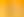What are the Chances?

# Stages1. Probability of an Event

EXPLORE

Suggested Learning Intentions

• To describe the probability of an event using words and numbers
• To understand the concepts of randomness and fairness and their impact on determining likelihood of events

Sample Success Criteria

• I can represent probability on a continuum from 0-1
• I can use the language of chance: certain, likely, equal chance, unlikely, impossible
• I can express probabilities using fractions, decimals, and percentages
• I can describe whether the probability of an event is random and/or fair
• I can use manipulatives to model my solution and thinking
EXPLORE

2. Theoretical and Experimental Probability

EXPLORE

Suggested Learning Intentions

• To describe probability in terms of what happened and what might be likely to happen in future events
• To compare the results of small and large trials to determine which provides a more accurate estimate of probability

Sample Success Criteria

• I can analyse a situation and describe probability in terms of what I expect to happen (theoretical)
• I can design and conduct an experiment, and collect and analyse data to determine how likely something will happen in the future (experimental)
• I can explain the ‘law of large numbers’
• I can apply my understanding of fractions and percentages to express probability of events
EXPLORE

3. Sample Space and Independence of Events

EXPLORE

Suggested Learning Intentions

• To analyse probability situations and predict the likelihood of events (independence of events)
• To list and compare all possible outcomes for an experiment or chance situation (sample space)
• To understand the difference between short-term variability and long-term stability (law of large numbers)

Sample Success Criteria

• I can identify dependant and independent events
• I can systematically list and count the possible outcomes in an experiment
• I can record probabilities using tallies, tables, and diagrams
• I can conduct repeated trials of chance experiments to determine the probability
• I can explain the notion of ‘in the long run...’ when determining probability
EXPLORE

4. Real World Probability

EXPLORE

Suggested Learning Intentions

• To analyse data to make predictions, answer questions and make decisions about real-world issues

Sample Success Criteria

• I can analyse and interpret data in context
• I can identify different factors that influence my interpretation of the data
• I can answer questions and make decisions based on data
• I can explain the certainty of my decisions
• I can model and justify my solutions using a range of manipulatives
EXPLORE